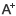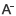# 高一数学常识点概括：集合日期：2019-12-16     来源：www.zhixueshuo.com    作者：智学网    浏览：540    评论：0

根据集合的概念，当一个集合的所有元素都已知时，这个集合就确定了。以下是我们为大家整理有关集合的高一数学常识点梳理，欢迎大家参阅!

高一数学常识点概括：集合

一.常识总结：

1.集合的有关定义。

1)集合：某些指定的对象集在一起就成为一个集合.其中每个对象叫元素

注意：①集合与集合的元素是两个不一样的定义，教科书中是通过描述给出的，这与平面几何中的点与直线的定义类似。

②集合中的元素具有确定性、互异性和无序性。

③集合具有两方面的意义，即：凡是符合条件的对象都是它的元素;只须是它的元素就需要符号条件

2)集合的表示办法：常用的有列举法、描述法和图文法

3)集合的分类：有限集，无限集，空集。

4)常用数集：N，Z，Q，R，N*

2.子集、交集、并集、补集、空集、全集等定义。

1)子集：若对xA都有xB，则A B;

2)真子集：A B且存在x0B但x0 A;记为A B

3)交集：AB={x| xA且xB}

4)并集：AB={x| xA或xB}

5)补集：CUA={x| x A但xU}

注意：①? A，若A?，则? A ;

②若 ， ，则 ;

③若 且 ，则A=B

3.弄清集合与元素、集合与集合的关系，学会有关的术语和符号，特别要注意以下的符号： 与 、?有哪些不同; 与 有哪些不同; 与 有哪些不同。

4.有关子集的几个等价关系

①AB=A A B;②AB=B A B;③A B C uA C uB;

④ACuB = 空集 CuA B;⑤CuAB=I A B。

5.交、并集运算的性质

①AA=A，A? = ?，AB=BA;②AA=A，A? =A，AB=BA;

③Cu = CuACuB，Cu = CuACuB;

6.有限子集的个数：设集合A的元素个数是n，则A有2n个子集，2n-1个非空子集，2n-2个非空真子集。

二.例题讲解：

【例1】已知集合M={x|x=m+ ,mZ},N={x|x= ,nZ},P={x|x= ,pZ}，则M,N,P满足关系

A) M=N P B) M N=P C) M N P D) N P M

剖析一：从判断元素的共性与不同入手。

解答一：对于集合M：{x|x= ,mZ};对于集合N：{x|x= ,nZ}

对于集合P：{x|x= ,pZ}，由于3+1和3p+1都表示被3除余1的数，而6m+1表示被6除余1的数，所以M N=P，故选B。

剖析二：容易列举集合中的元素。

解答二：M={， ，}，N={， , , ，}，P={， , ，}，这个时候不要急于判断三个集合间的关系，应剖析各集合中不一样的元素。

= N， N，M N，又 = M，M N，

= P，N P 又 N，P N，故P=N，所以选B。

点评：由于思路二只是停留在最初的总结假设，没有从理论上解决问题，因此提倡思路一，但思路二易人手。

变式：设集合 ， ，则

A.M=N B.M N C.N M D.

解：当 时，2k+1是奇数，k+2是整数，选B

【例2】概念集合A*B={x|xA且x B}，若A={1,3,5,7},B={2,3,5}，则A*B的子集个数为

A)1 B)2 C)3 D)4

剖析：确定集合A*B子集的个数，第一要确定元素的个数，然后再借助公式：集合A={a1，a2，，an}有子集2n个来求解。

解答：∵A*B={x|xA且x B}， A*B={1,7}，有两个元素，故A*B的子集共有22个。选D。

变式1：已知非空集合M {1,2,3,4,5}，且若aM，则6?aM，那样集合M的个数为

A)5个 B)6个 C)7个 D)8个

变式2：已知{a,b} A {a,b,c,d,e},求集合A.

解：由已知，集合中需要含有元素a,b.

集合A可能是{a,b},{a,b,c},{a,b,d},{a,b,e},{a,b,c,d},{a,b,c,e},{a,b,d,e}.

评析 本题集合A的个数实为集合{c,d,e}的真子集的个数，所以共有 个 .

【例3】已知集合A={x|x2+px+q=0},B={x|x2?4x+r=0},且AB={1},AB={?2,1,3},求实数p,q,r的值。

解答：∵AB={1} 1B 12?41+r=0,r=3.

B={x|x2?4x+r=0}={1,3}, ∵AB={?2,1,3},?2 B, ?2A

∵AB={1} 1A 方程x2+px+q=0的两根为-2和1，

变式：已知集合A={x|x2+bx+c=0},B={x|x2+mx+6=0},且AB={2},AB=B，求实数b,c,m的值.

解：∵AB={2} 1B 22+m?2+6=0,m=-5

B={x|x2-5x+6=0}={2,3} ∵AB=B

又 ∵AB={2} A={2} b=-=4,c=22=4

b=-4,c=4,m=-5

【例4】已知集合A={x|0},集合B满足：AB={x|x-2}，且AB={x|1

剖析：先化简集合A，然后由AB和AB分别确定数轴上哪些元素属于B，哪些元素不属于B。

解答：A={x|-21}。由AB={x|1-2}可知[-1,1] B，而B=ф。

综合以上各式有B={x|-1x5}

变式1：若A={x|x3+2x2-8x0}，B={x|x2+ax+b0},已知AB={x|x-4}，AB=,求a,b。

点评：在解有关不等式解集一类集合问题，应注意用数形结合的办法，作出数轴来解之。

变式2：设M={x|x2-2x-3=0},N={x|ax-1=0}，若MN=N，求所有满足条件的a的集合。

解答：M={-1,3} , ∵MN=N, N M

①当 时，ax-1=0无解，a=0 ②

综①②得：所求集合为{-1，0， }

【例5】已知集合 ，函数y=log2的概念域为Q，若PQ，求实数a的取值范围。

剖析：先将原问题转化为不等式ax2-2x+20在 有解，再借助参数分离求解。

解答：若 ， 在 内有有解

令 当 时，

所以a-4,所以a的取值范围是

变式：若关于x的方程 有实根,求实数a的取值范围。

解答：

点评：解决含参数问题的题目，一般要进行分类讨论，但并不是所有的问题都要讨论，如何可以防止讨论是大家考虑此类问题的重要。

0相关评论